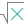#MULTINOMIAL (PQL - xl)

This function calculates the ratio of the factorial of a sum of values to the product of factorials

• Library: PQL \ Spreadsheet \ Math & Trig
• Compatibility: Any content (regardless of data source) in the Tabulate spreadsheet module
• Solve: This function can be used with the Solve plug-in: both as part of the objective function and constraint functions

#### Syntax

MULTINOMIAL(number1, number2)

##### Function Arguments
 Name Description Type Optional number1 values to calculate in the multinomial. Each argument can be a double-precision floating point value, an integer value, or an array of these StringOrNumber number2 values to calculate in the multinomial. Each argument can be a double-precision floating point value, an integer value, or an array of these StringOrNumber